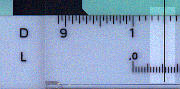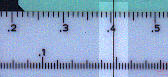Exponentiation

Introduction

In many branches of science it is necessary to raise a non-integer number to a non-integer power.

An example from hydrology is the estimate of the world's largest observed rainfall events which is given by the formula:

R = 426 * D 0.475

where:
R is rainfall in millimetres
D is duration in hours.

Another example, from the world of finance, relates to compound interest where the capital accrued is given by:

C = I * (1 + r/100) P

where:
C is capital accrued at the end of the period.
I is the initial investment
r is the rate of interest (%)
P is the period (years)

There are in fact four different types of calculations of the form c = ab

 a b c >1.0 >1.0 c>1.0: c> a >1.0 <1.0 c>1.0: c1.0 c<1.0: ca

We will consider each type in turn.

For most of the examples we present are using a Graphoplex 690a. This French made rule has very clear markings but for some of the calculations we will also consider other rules which have different scale arrangements.

These calculations are performed using the log-log scales. Typically a rule may have three log-log scales labelled LL1 to LL3 covering the following ranges.
LL1: 1.01 to 1.11
LL2: 1.10 to 3.0
LL3: 2.5 to 500,000.
In this case the scales extend slightly beyond the length of the normal scales which gives some overlap in the values. These scale are used for values greater than one.

One many rules you will also find scales labelled LL01 to LL03. These typically cover the ranges:
LL01: 0.99 to 0.90
LL02: 0.91 to 0.35
LL03: 0.39 to .00002
If your rule does not have scales for these ranges of values less than one you can calculate the reciprocal of the value, perform the calculation using the log-log scales for numbers greater than one and then take the reciprocal of the answer.

Some rules, including this one, have scales labelled LL0 and LL00 for numbers very close to 1.

Other rules have scales which cover different ranges. For example the Pickett N4-T has the following scales:
LL1: 1.0025 to 1.023
LL2: 1.025 to 1.25
LL3: 1.25 to 10.0
LL4: 10.0 to 109

This rule actually uses logarithms to a base of 10 whereas most rule use logarithms to a base of e (2.72). The scale arrangement has implications for accuracy of calculation but not for the way in which the scales are used. It also has the scales for numbers less than one alongside the scales for numbers greater than one. This saves space and does seem to make the rule more difficult to use.

On most rules the log-log scales are on the stock but one variation you also sometimes find is to have the log-log scales on the slide rather than the stock. This is also described below.

Finally we should note an important difference between log-log scales and many other slide rule scales. With many slide rule calculations the position of the decimal point does not necessarily correspond to that marked on the scale. For calculations involving the log-log scales this is not the case. The value on the log-log scale has the value shown and no other.

Example 1: a >1, b > 1

Let us consider 1.05 7.0

Cursor to 1.05 on LL1
1 on C to cursor
Cursor to 7 on C
Answer (1.407) under cursor on LL2.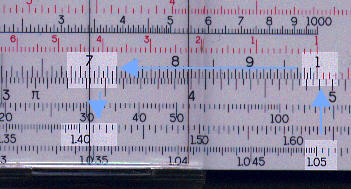Note that in this case although we started on LL1, the answer "moved up" a scale to LL3.

Example 2: a >1, b < 1

Let us consider 7.56 0.21. The method is very similar to the above example:

Cursor to 7.56 on LL3
1 on C to cursor
Cursor to .21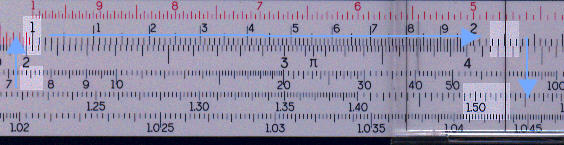Example 3: a<1, b>1

Let us consider 0.5 1.5

Cursor to 0.5 on LL02
1.5 on CI to cursor.
(This is variant which can also be applied to the above. We have used the CI scale in a similar way to which it can be used for multiplication.)
Cursor to 1.0 on C scale
Answer (0.353) on LL02 under cursor.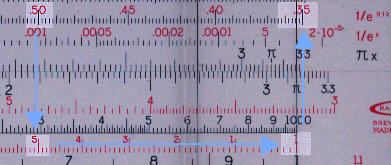Example 4: a<1, b<1

Let us consider 0.92 0.75

Cursor to 0.92 on LL01
1 on C to cursor
Cursor to .75 on C
Answer (.9394) under cursor on LL01.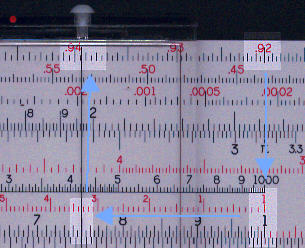Calculations for numbers less than one when LL0 scales are not present

Let us consider 0.92 0.75, one of the examples above. As the calculation is very similar to the above we do not present images.

1/0.92 = 1.087
Cursor to 1.087 on LL1
1 on C to cursor
Result (1.064) under cursor.

As can be seen this is much more cumbersome, the values cannot be transferred directly from the log-log scales to the C and D scales for calculating the reciprocal, and there is a loss of accuracy.

Calculation with log-log scales on slide

Let us consider 1.05 7.0, which was our first example.

Cursor to 1 on C
1.05 on LL1 to cursor
Cursor to 7 on C
Answer (1.407) under cursor on LL2.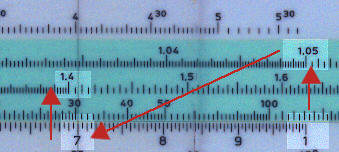Use of logarithm scale when no log-log scales are present

Even without log-log scales it is possible to calculate expressions using non-integer powers. The basic method is:

ab = 10 (b * log a ), where the logarithms are to base 10.

To do this you need a scale of logarithms which is  more commonly found than log-log scales.

Let us consider 1.05 7.0 , an example used earlier.

Cursor to 1.05 on C
Read the logarithm of 1.05 (.021) on L
Multiply .021 by 7.0 = .147. (Not illustrated)
Cursor to .147 on L.